Friday, October 22, 2021
Home > CBSE Class 10 > Real Numbers CBSE Class 10 MCQ Questions with Answers Keys

# Real Numbers CBSE Class 10 MCQ Questions with Answers KeysHi students, Welcome to AMBiPi (Amans Maths Blogs). In this article, you will get Real Numbers CBSE Class 10 MCQ Questions with Answer Keys. You can download this PDF and save it in your mobile device or laptop etc.

Real Numbers CBSE Class 10 MCQ Question No 41:

If a and b are two odd prime numbers such that a > b, then a2 – b2 is

Option A : an even number

Option B : an odd number

Option C : an odd prime number

Option D : a prime number

Option A : an even number

Real Numbers CBSE Class 10 MCQ Question No 42:

Irrational number between 2 and 2.5 is

Option A : √2.2

Option B : √5

Option C : √2

Option D : √2.5

Option B : √5

Real Numbers CBSE Class 10 MCQ Question No 43:

The rational form ofis in the form of p/q, then (p + q) is

Option A : 14

Option B : 55

Option C : 69

Option D : 79

Option C : 69

Real Numbers CBSE Class 10 MCQ Question No 44:

If x = 7 + 4√3, then the value of √x + 1/√x is

Option A : 6

Option B : 4

Option C : 2√3

Option D : 4√3

Option B : 4

Real Numbers CBSE Class 10 MCQ Question No 45:

The rational number of the form p/q, where q ≠ 0, p and q are positive integers, which represents, i.e. (0.1343434 ……….) is

Option A : 134/999

Option B : 134/990

Option C : 133/999

Option D : 133/990

Option D : 133/990

Real Numbers CBSE Class 10 MCQ Question No 46:

Which one of the following numbers can be represented as non-terminating, repeating decimals

Option A : 39/24

Option B : 3/16

Option C : 3/11

Option D : 137/25

Option D : 137/25

Real Numbers CBSE Class 10 MCQ Question No 47:

If x =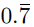, then 2x is

Option A :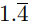Option B :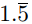Option C :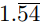Option D :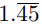Option B :

Real Numbers CBSE Class 10 MCQ Question No 48:

Find the remainder when 27 × 43 × 46 is divided by 10.

Option A : 1

Option B :

Option C : 3

Option D : None of these

Option B : 6

Real Numbers CBSE Class 10 MCQ Question No 49:

A number in the form of 15q + 7 can be written as

Option A : 3m + 3

Option B : 5m + 2

Option C : 5m + 3

Option D : 3m + 2

Option B : 5m + 2

Real Numbers CBSE Class 10 MCQ Question No 50:

The least number which is a perfect square and is divisible by each of 16, 20 and 24 is

Option A : 240

Option B : 1600

Option C : 2400

Option D : 3600